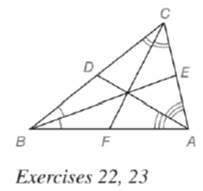Chapter 5.6, Problem 23E### Elementary Geometry for College St...

6th Edition
Daniel C. Alexander + 1 other
ISBN: 9781285195698

#### Solutions

Chapter
Section### Elementary Geometry for College St...

6th Edition
Daniel C. Alexander + 1 other
ISBN: 9781285195698
Textbook Problem
1 views

# In Δ A B C , A C = 5.3 , B C = 7.2 and B A = 6.7. With angle bisectors as shown, which line segment is longer?a) A E ¯ or E C ¯ ? b) C D ¯ or D B ¯ ? c) A F ¯ or F B ¯ ?

To determine

a)

To find:

Which line segment is longer

AE¯ or EC¯?

Explanation

Angle-Bisector Theorem:

If a ray bisects one angle of a triangle, then it divides the opposite side into segments whose lengths are proportional to the lengths of the two sides that form the bisected angle.

Calculation:

Given,

AC=5.3,BC=7.2andBA=6.7

From Angle-Bisector theorem,

In ΔABC

ECBC=EABA.........(1)

Let EC=x,

EA=CACE......(2)

Substitute EC=x and AC=5.3 in equation (2) we get,

EA=5.3x

Substitute EA=5.3x,BC=7.2andBA=6

To determine

b)

To find:

Which line segment is longer

CD¯ or DB¯

To determine

c)

To find:

Which line segment is longer

AF¯ or FB¯

### Still sussing out bartleby?

Check out a sample textbook solution.

See a sample solution

#### The Solution to Your Study Problems

Bartleby provides explanations to thousands of textbook problems written by our experts, many with advanced degrees!

Get Started

#### Find more solutions based on key concepts